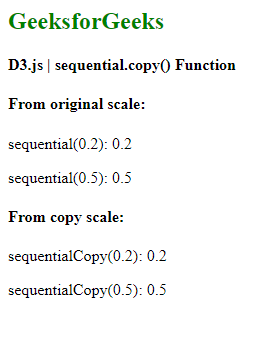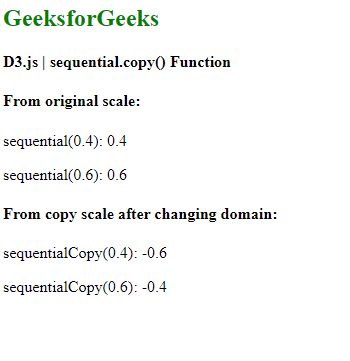# D3.js sequential.copy() Function

• Last Updated : 31 Aug, 2020

The sequential.copy() function in D3.js is used to construct and return a copy of the original scale. Any changes in original scale will not affect the copy scale and vice-versa. Sequential scales are similar to continuous scales. In a continuous scale, mapping is done in a continuous way between domain and range. The only difference is that the output range of this scale is fixed by its interpolator and this range cannot be changed.

Syntax:

`sequential.copy()`

Parameters: This function does not accept any parameters.

Return Values: This function returns the copy of the original scale.

Below given are a few examples of sequential.copy() in D3.js:

Example 1:

## HTML

 ```<``html``>`` ` `<``head``>``    ``<``script` `src``=``"https://d3js.org/d3.v6.min.js"``>``    `````` ` `<``body``>``    ``<``h2` `style``=``"color:green"``>``        ``GeeksforGeeks``    ```` ` `    ``<``h4``> D3.js | sequential.copy() Function ``     ` `    ``<``script``>``        ``var sequential = d3.scaleSequential();`` ` `        ``// Default scale is identity function``        ``document.write("<``h4``>From original scale:")`` ` `        ``document.write("<``p``>sequential(0.2): ", ``                    ``sequential(0.2) + "");``         ` `        ``document.write("<``p``>sequential(0.5): ", ``                    ``sequential(0.5) + "");`` ` `        ``// Creating copy of the original scale``        ``var sequentialCopy = sequential.copy();``        ``document.write("<``h4``>From copy scale:")``        ``document.write("<``p``>sequentialCopy(0.2): ", ``                    ``sequentialCopy(0.2) + "");`` ` `        ``document.write("<``p``>sequentialCopy(0.5): ", ``                    ``sequentialCopy(0.5) + "");``    `````` ` ``

Output:Example 2: Any change in the copy scale does not affect the original scale.

## HTML

 ```<``html``>`` ` `<``head``>``    ``<``script` `src``=``"https://d3js.org/d3.v6.min.js"``>``    `````` ` `<``body``>``    ``<``h2` `style``=``"color:green"``>``        ``GeeksforGeeks``    ```` ` `    ``<``h4``> D3.js | sequential.copy() Function `` ` `    ``<``script``>``        ``var sequential = d3.scaleSequential();`` ` `        ``// Default scale is identity function``        ``document.write("<``h4``>From original scale:");`` ` `        ``document.write("<``p``>sequential(0.4): ", ``                    ``sequential(0.4) + "");``        ``document.write("<``p``>sequential(0.6): ", ``                    ``sequential(0.6) + "");`` ` `        ``// Creating copy of the original scale``        ``var sequentialCopy = sequential.copy();``        ``sequentialCopy.domain([1, 2]);``        ``document.write(``        ``"<``h4``>From copy scale after changing domain:");`` ` `        ``document.write("<``p``>sequentialCopy(0.4): ", ``                    ``sequentialCopy(0.4) + "");``        ``document.write("<``p``>sequentialCopy(0.6): ", ``                    ``sequentialCopy(0.6) + "");``    `````` ` ``

Output:My Personal Notes arrow_drop_up